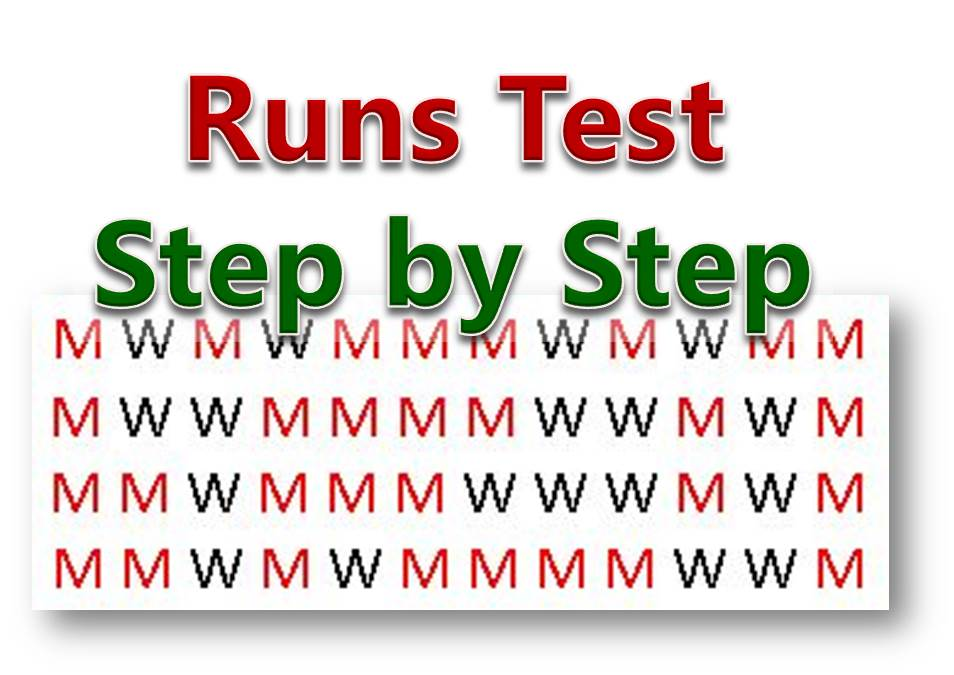# Hypothesis Testing Question 21 – Wald-Wolfowitz Run Test for Large Sample (Step by Step Procedure)This is how to Perform Wald-Wolfowitz Run Test

Question 21
The following arrangement of men, M, and women, W, lined up to purchase tickets for a rock concert:

Test for randomness at the α = 0.05 significance level

Solution Steps
In this case, we see that the sample size is fairly large, so we are going to use a slightly different method in this case. We are going to calculate the mean runs and the standard deviation.

Then we go ahead to find the z-statistic. We would use the following 7 steps to disolve this problem.

### Step 1: State the null and alternate hypothesis

H0: Arrangement is random
H1: Arrangment is not random

### Step 2: Determine the Number of Runs

This means that you need to mark each of the categories so you can easily count them
Here I mark each run alternatively with red for M and black wor W. The outcome is given below.

The number of runs is given by R = 27
The number of Men, n1 = 30
The number of Women, n2 = 18

### Step 3: Calculate the Mean runs

The mean is given by the formula

We can go ahead to substitute the value of n1 = 30 and n2 = 18

### Step 4: Calculate the Standard Deviation

You can  find the standard deviation for a runs test using the formula

We can go ahead to substitute the value of n1 = 30 and n2 = 18

### Step 5: Calculate the z-Statistic

The z-Statistic can be calculated using the formula

Then we can substitute the value of
R = 27
μR = 23.5
σR = 3.2083

### Step 6: Determine the Critical Value

Look up the value of the critical value from statistical table of normal distribution
We get a critical value for of 1.96

### Step 7: State the Decision

Since the calculated value of z = 1.0909 is within the accept region(less than the critical value of 1.96), we therefore accept (fail to reject) the null hypothesis and conclude that there is not real evidence that the arrangement is not random